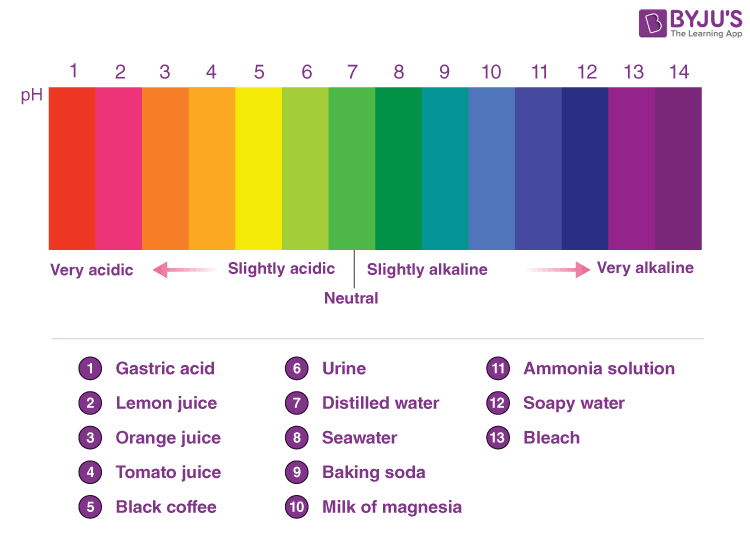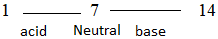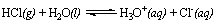Win up to 100% scholarship on Aakash BYJU'S JEE/NEET courses with ABNAT Win up to 100% scholarship on Aakash BYJU'S JEE/NEET courses with ABNAT

# pH Scale and Acidity

The pH scale is a commonly used scale to measure the acidity or the basicity of a substance. The possible values on the pH scale range from 0 to 14.  Acidic substances have pH values ranging from 1 to 7 (1 being the most acidic point on the pH scale), and alkaline or basic substances have pH values ranging from 7 to 14. A perfectly neutral substance will have a pH of exactly 7.

pH, which is an abbreviation of the ‘potential for hydrogen’ or ‘power of hydrogen’ of a substance, can be expressed as the negative logarithm (with base 10) of the hydrogen ion concentration in that substance. Similarly, the pOH of a substance is the negative logarithm of the hydroxide ion concentration in the substance. These quantities can be expressed via the following formulae:

• pH = -log[H+]
• pOH = -log[OH]

It is important to note that the pH scale is a logarithmic scale. Therefore, an increase in the pH value of a solution by one will be accompanied by a tenfold increase in the hydrogen ion concentration and, therefore, a tenfold increase in acidity. Elaborating with the help of an example, a solution with a pH of 3 will have ten times the acidity of a solution with a pH of 4 and a hundred times the acidity of a solution with a pH of 5. On the other hand, we can say that pH is a dimensionless quantity.

## Introduction to pH Scale

The pH scale is mostly attributed to a set of chosen solutions whose pH is already established by the international committee. Alternatively, the primary pH standard values are determined using a concentration cell with transference, wherein the potential difference is measured between a hydrogen electrode and a standard electrode. Typically, the pH of an aqueous solution is measured with the help of a glass electrode or a pH meter. A colour-changing indicator is also used. In any case, pH measurement and pH scale are quite essential in various fields of Chemistry, Medicine, Water Treatment and other applications.### pH Scale Properties

• The pH scale is very convenient to use. In this scale, odd expressions such as 1.24 × 10-4 are converted into a single number of 3.89.
• This scale covers a very large range of [H+][H+].
• The division between zero and 1 is expanded to a linear scale. The pH scale also expands the compact scale into a large scale for comparison purposes.
• As there is a use of a negative log of [H+][H+] in the scale, the pH scale actually has positive values. It should be noted that if the pH is large, the [H+][H+] will be smaller.
• The pH range does not have any lower or upper bound. It is because the pH is an indication of the concentration of H+.

### Unified Absolute pH Scale

In the year 2010, a new unified absolute pH scale was proposed. As per the details mentioned, this scale would allow various pH ranges across different solutions to be measured based on a common proton reference standard. This was to be developed on the basis of the absolute chemical potential of the proton. Additionally, this model used the Lewis acid-base definition and has been applied to liquids, gases and even solids.

## pH Scale and Acidity

Acid solutions usually have protons, and basic solutions have hydroxide ions. The concentrations of the ions are low (negative power of ten). pH scale is a convenient way of expressing these low concentrations in simple numbers between 1 and 14.

pH is the negative logarithm to the base ten of hydrogen ion concentration in moles per litre.

pH = – log [H+]

p(OH) is the negative logarithm to the base ten of hydroxide ion concentration in moles per litre.

p(OH) = – log [OH]

In aqueous solutions, pH + p(OH) = 14.

pH scale is based on neutral water, where [H+] = [OH] = 10-7

For a neutral solution pH = = – log [H+] = – log [10-7] = +7

pH of strong acid decreases with a limit of 1, and the pH of a base increases up to 14.

Generally, acids and bases will have a pH betweenBut negative and greater than 14 pH values are also possible.

Also Read: Study the pH Change

## Limitations of pH Scale

1. pH values do not reflect directly the relative strength of acids or bases. A solution of pH = 1 has a hydrogen ion concentration 100 times that of a solution of pH = 3 (not three times). A 4 x 10-5 N HCI is twice concentrated as a 2 x 10-5 N HCI solution, but the pH values of these solutions are 4.40 and 4.70 (not double).

2. The pH value is zero for 1 N solution of strong acid. The concentration of 2 N, 3 N, 10 N, etc., gives negative pH values.

3. A solution of an acid having a very low concentration, say 10-8 N, shows a pH value of 8, and hence should be basic, but the actual pH value is less than 7.

4. A range of 0 to 14 provides sensible (but not absolute) measures for the scale. There are cases when we can go below zero and sometimes also go above 14 in water, as the concentrations of hydronium ions or hydroxide ions can go beyond one molar.

## Periodic Variation of Acidic and Basic Properties

(a)  Hydracids of the Elements of the Same Periods

Along the period, acidic strength increases. Hydrides become increasingly acidic from CH4, NH3, H2O and HF. The increase in acidic properties is due to the fact that the stability of their conjugate bases increases in the order

CH3< NH2  < OH– < F

(b)  Hydracids of the Elements of the Same Group

1. The acidic nature increases down the column. Hydrides of V group elements (NH3, PH3, AsH3, SbH3, BiH3) show basic character, which decreases due to an increase in size and a decrease in electronegativity from N to Bi. There is a decrease in electron density in the sp-hybrid orbital, and thus electron donor capacity decreases.
2. Hydracids of VI group elements (H20, H2S, H2Se, H2Te) act as weak acids. The strength increases in the order H20 < H2S < H2Se < H2Te. The increasing acidic properties reflect the decreasing trend in the electron donor capacity of OH, HS, HSe or HTe ions.
3. Hydracids of VII group elements (HF, HCI, HBr, HI) show acidic properties, which increase from HF to HI. This is explained by the fact that bond energies decrease.

(H-F = 135 kcal/mol, HCI = 103, HBr = 88 and HI = 71 kcal/mol).

## Oxyacids

The acidic properties of oxyacids of the same element, which are in different oxidation states, increase with an increase in oxidation number.

+ 1            +3          +5            +7

HCIO  <  HC1O2   <   HC1O3   <  HCIO4

+4            +6         +3            +5

H2SO3  <   H2SO4;    HNO2     <  HNO3

But this rule fails in oxyacids of phosphorus.

H3PO2  >  H3PO3  >  H3PO4

The acidic properties of the oxyacids of different elements which are in the same oxidation state decrease as the atomic number increases. This is due to an increase in size and a decrease in electronegativity.

HC1O4 > HBrO4 > HIO4

H2SO3  > H2SeO3

But there are a number of acid-base reactions in which no proton transfer takes place.

For example,

SO+ SO2  ↔  SO2+ +  S

Acid1  Base2    Acid2   Base1

Thus, the protonic definition cannot be used to explain the reactions occurring in non-protonic solvents such as COCl2, S02, N2O4, etc.

## Water – Amphoteric Weak Electrolyte

1) Water can behave like an acid or a base. So, it is amphoteric.Water accepts protons from HCl and acts as a base.

Water gives proton to ammonia and can be an acid

$$\begin{array}{l}NH_3(aq)+H_2O(l)\rightleftharpoons NH_{4}^{+}(aq)+OH^{-}\end{array}$$

### Molarity of Water

Molarity = Number of moles per litre of solution = = 55.55 mole l-1

### Ionization Constant of Water

$$\begin{array}{l}H_2O\rightleftharpoons H_{4}^{+}+OH^{-}\end{array}$$
$$\begin{array}{l}Ka = Kb = \frac{[H+[OH-]]}{[H_2O]}\end{array}$$
$$\begin{array}{l}=\frac{10^{-7}\times 10^{-7}}{55.55}=1.8\times10^{-16}\end{array}$$

Where, Ka is the acid ionization constant, and Kb is the base ionization constant.

pKa = pKb = – log[Ka]

$$\begin{array}{l}=-\log 1.8\times10^{-16}\end{array}$$
= 15.74

### Degree of Ionization of Water

$$\begin{array}{l}H_2O\rightleftharpoons H^{+}+OH^{-}\end{array}$$

Initial concentration moles 55.55 0 0

At equilibrium moles 10-7 10-7

Degree of ionization = α

$$\begin{array}{l}=\frac{number\;of\;moles\;ionized}{initial\;number\;of\;moles}\end{array}$$
$$\begin{array}{l}=\frac{10^{-7}}{55.55}=1.8\times 10^{-9}\end{array}$$

Only about 2 parts per billion (ppb) of the water molecules dissociate into ions at room temperature.

### Ionic Product of Water

It is the product of the concentrations of hydrogen and hydroxide ions in water.

Ionic product of water = Kw = [H+][OH] = 10-14

pKw = – log[Kw] = – log10-14= 14

Ionic product, pKw, pKa and pKb remains the same whether the solution is acidic, neutral or basic.

Related Topics

Test Your Knowledge on pH Scale and Acidity